Home > GB8I > Chapter cc46 > Lesson cc46.3.3 > Problem6-108

6-108.
1. Examine the graphs below. Decide if each graph represents a function. Then describe the domain and range of each one. Homework Help ✎

2.  a.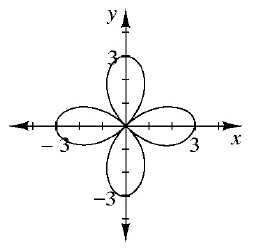b.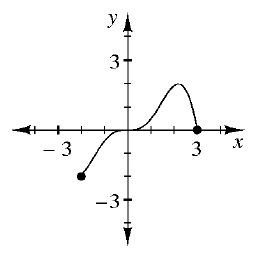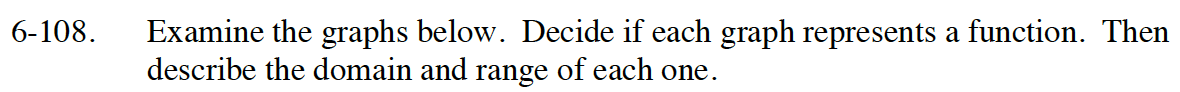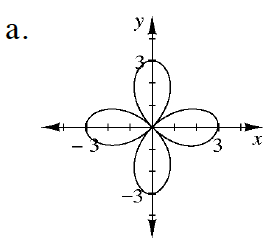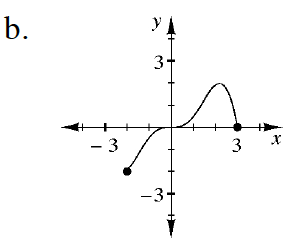Is there more than one output value for a single input value?
If so, it is not a function.

Determine the possible x-values to find the domain and the possible y-values to find the range.

It is not a function.
R: − 3 ≤ x ≤ 3

Is there more than one output value for a single input value?
If so, it is not a function.

Determine the possible x-values to find the domain and the possible y-values to find the range.

It is a function.
D: −2 ≤ x ≤ 3